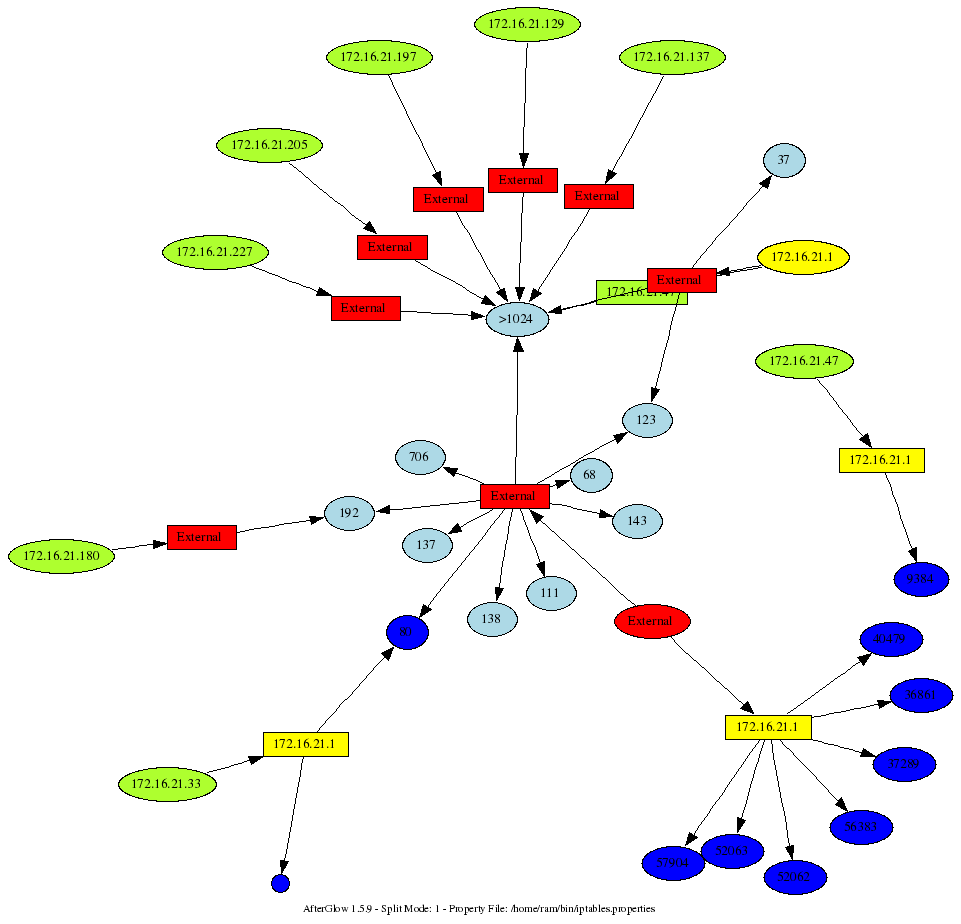June 18, 2007

## AfterGlow Example – Visualizing IP Tables Logs

Category: Log Analysis,Visualization — Raffael Marty @ 11:13 am

I am sitting in Seville, at the First conference, where I will be teaching a workshop on Wednesday. The topic is going to be insider threat visualization. While sitting in some of the sessions here, I was playing with my iptables logs. Here is a command I am running to generate a graph from my iptables logs:
`cat /var/log/messages | grep "BLOCK" | perl -pe 's/.*\] (.*) (IN=[^ ]*).*(OUT=[^ ]*).*SRC=([^ ]* ).*DST=([^ ]* ).*PROTO=([^ ]* ).*?(SPT=[^ ]+ )?.*?(DPT=\d* )?.*?(UID=\d+)?.*?/\1,\2,\3,\4,\5,\6,\7,\8,\9/' | awk -F, '{printf "%s,%s,%s\n",\$4,\$5,\$8}' | sed -e 's/DPT=//' -e 's/ //g' | afterglow.pl -e 1.3 -c iptables.properties -p 1 | neato -Tgif -o/tmp/test.gif; xloadimage /tmp/test.gif`
This is fairly ugly. I am not going to clean it up. You get the idea. Now, what does the iptables.properties look? Well, if you thought the command above was ugly. The properties file is completely insane, but it shows you the power of using the “variable” assignment:
``` variable=\$ip=`ifconfig eth1 | grep inet` variable=\$ip=~s/.*?:(\d+\.\d+\.\d+\.\d+).*\n?/\1/; variable=\$subnet=\$ip; \$subnet=~s/(.*)\.\d+/\1/; variable=\$bcast=\$subnet.".255"; ``````color="invisible" if (field() eq "\$bcast") color="invisible" if (\$fields eq "67") color="yellow" if (field() eq "\$ip") color.source="greenyellow" if (\$fields=~/^192\.168\..*/); color.source="greenyellow" if (\$fields=~/^10\..*/); color.source="greenyellow" if (\$fields=~/^172\.16\..*/); color.source="red" color.event="greenyellow" if (\$fields=~/^192\.168\..*/) color.event="greenyellow" if (\$fields=~/^10\..*/) color.event="greenyellow" if (\$fields=~/^172\.16\..*/) color.event="red" cluster.target=">1024" if ((\$fields>1024) && (\$fields ne "\$ip")) cluster.source="External" if (field()!~/^\$subnet/) cluster.event="External" if (field()!~/^\$subnet/) color.target="blue" if (\$fields eq "\$ip") color.target="lightblue"```
Mind you, this was run on an Ubuntu Linux. You might have to change some of the commands and parsing of the output. Pretty neat, ey? Here is a sample output that this generated. My box is yellow. The question that I was trying to answer: Why is someone trying to conect to me on port 80?Technorati Tags: , , ,